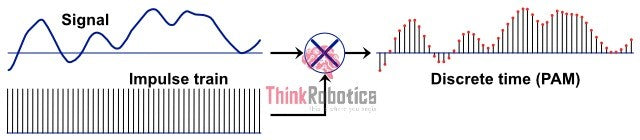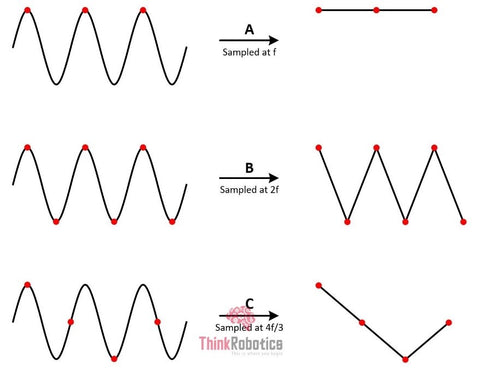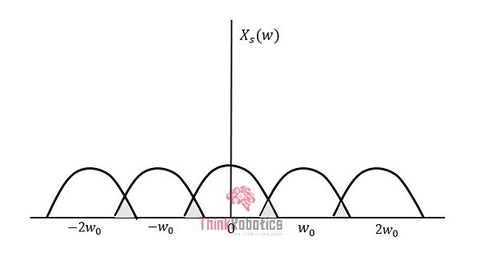Free Shipping for orders over ₹1999

support@thinkrobotics.com | +91 93183 94903# Sampling of Signal | ThinkRobotics.in

Our world is filled with signals, the vibration due to the running of any motor or any audio/video signal. All of these real-world signals are analog signals and vary continuously concerning time.

An analog signal is a signal that is entirely free to vary its amplitude from -∞ to +∞ , i.e., it can take any value on the amplitude axis. At the same time, digital signals take discrete values on the amplitude axis, which is usually the Y-axis. The worldly examples of signals are mostly continuous time-type, meaning the signal has no break-point or is continuous throughout its life.

However, digital computers and computer programs cannot process these continuous real-time signals. Thus, there rises a necessity to convert them to discrete-time signals.

Thus, converting a signal from continuous-time to discrete-time is called the Sampling of The Signal. In this process - the value of the signal is measured at certain intervals in time. Each measurement is referred to as a sample.

The Higher the number of such samples higher is the precision of its replication.

The below figure specifies a continuous-time signal and a sampled signal. When the original signal is multiplied by a periodic impulse train, the discrete-time signal is obtained.

An impulse train is a sum of shifted impulses.Fig 1: the process of Sampling

When the continuous-time signal is sampled at some frequency, say F, the resultant discrete signal has more frequency components than the original signal. To be accurate, the frequency components of the continuous-time signal are repeated at the sample rate. The discrete frequency response is seen at their original position and are also seen centered around +/- F, and around +/- 2F, etc.

The Sampling rate represents the number of samples taken per second or for a finite period.

Rate = 1 = Fs / Ts

Where Ts is the sampling time and fs is the sampling rate or the sampling frequency.

The Nyquist Rate: Now, how many such samples will help us the best in recreating the original signal and preserving most of it? In general, to preserve the signal's full information, it is necessary to sample at twice the maximum frequency of the signal. This is known as the Nyquist rate.

The Sampling Theorem: The Sampling Theorem states that” a continuous time signal can be represented in terms of its samples and can be recovered back when sampling frequency fs is greater than or equal to the twice the highest frequency component of message signal”.

The sampling theorem or the Nyquist-Shannon Sampling Theorem-

Fs > 2*FmaxFig 2: Sampling at various frequencies

If this condition satisfies, the analog signal is correctly represented in discrete form. The other analog signal may be losing its amplitude values for specific time intervals.

So, now we know that if the Nyquist Criteria is met, then the signals are sampled in the best manner, and their retrieval in the future becomes easy and efficient.

Now let us consider 3 cases; that is what happens when the signal is sampled at twice the highest frequency, at less than twice the highest frequency, and greater than twice the original message signal's highest frequency.

#### Fs = 2 * Fmax:

If the signal is sampled exactly twice the highest frequency component of the message signal by following the Sampling theorem, the signal is expected to retain all the information and data while undergoing the conversion. Moreover, the recovery of statistics or further conversion does not lead to its loss, provided the same is followed as and when needed. This is the Nyquist rate. It is the critical rate of sampling.Fig 3: Sampling at Nyquist rate

#### Fs > 2 * Fmax:

If the signal is sampled at a higher rate than 2fmax, then the information is reproduced without any loss. There is no mixing up and hence recovery is possible.Fig 4:Sampling at higher frequency

#### Fs < 2 * Fmax:

When sampled at this rate, there is a big chance that the information is lost. The overlapping of signals is observed here. This phenomenon of signal overlapping, as mentioned earlier, is called Aliasing. It is an effect that causes various signals to become indistinguishable when sampled. It also makes the reconstruction of the original signal almost an impossible task. That is, the recovered signal (continuous-time signal) might contain unwanted components.Fig 5: Sampling at lesser frequency
Where w = 2 * ∏ * frequency in the figures.

### Where do we use this technique?Fig 6: depiction of audio sampling process
1. Audio Sampling: The stored audio signals are in the digital form equivalent to the discrete form of the recorded initially continuous signal captured from acoustic events/sources.
1. Speech Sampling: Signals that carry human speech are usually be sampled at a much lower rate. Almost all of them vary in the frequency range of 100 Hz–4 kHz range, allowing a sampling rate of 8 kHz.
2. Video Sampling: Video digital-to-analog converters operate in the megahertz range from about 3 MHz for low-quality composite video scalers in early games consoles to 250 MHz or more for the highest-resolution VGA output.
3. 3D Sampling: The process of volume rendering samples a 3D grid of voxels (a voxel is a unit of visual information that defines a point in 3-D) to produce 3D renderings of sliced (tomographic) data. The 3D grid denotes a continuous region of 3D space. Volume rendering is standard in medical imaging; X-ray computed tomography (CT/CAT), magnetic resonance imaging (MRI), positron emission tomography (PET) are some examples.
1. Radar Signal Processing: Using sampling technology, the radar signal is transformed at the signal's receiving end. Also, the signal processing involves inhibition of multiplicative noise and additive noise; thus, more practical radar signals are obtained.

Thus, sampling of signals is a prevalent technique used in many instruments like analog-to-digital converters, etc., even the cellular calling system uses this.Fig 7: Volume rendering and reconstruction

Sources: